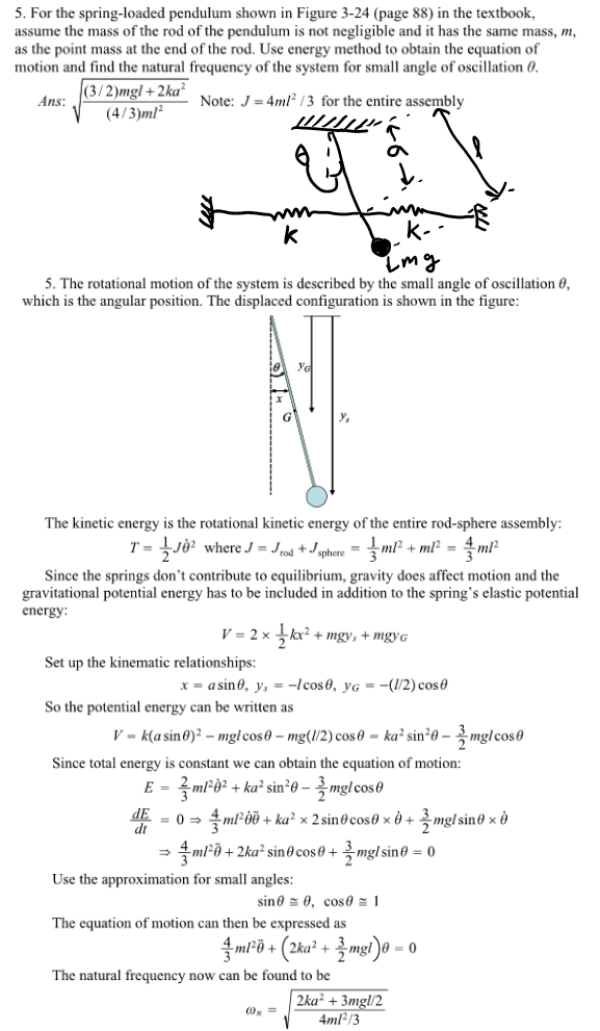For the spring-loaded pendulum shown in the figure in the textbook, assume the mass of the rod of the pendulum is not negligible and it has the same mass, m, as the point mass at the end of the rod. Use energy method to obtain the equation of motion and find the natural frequency of the system for small angle of oscillation theta.For the spring-loaded pendulum shown in the figure in the textbook, assume the mass of the rod of the pendulum is not negligible and it has the same mass, m, as the point mass at the end of the rod. Use energy method to obtain the equation of motion and find the natural frequency of the system for small angle of oscillation theta.

System Dynamics Page 2 dynamics dynamics dynamics dynamics dynamics dynamics dynamics System dynamics Page 3